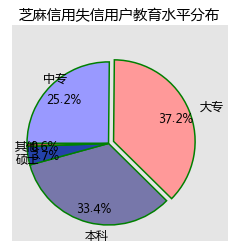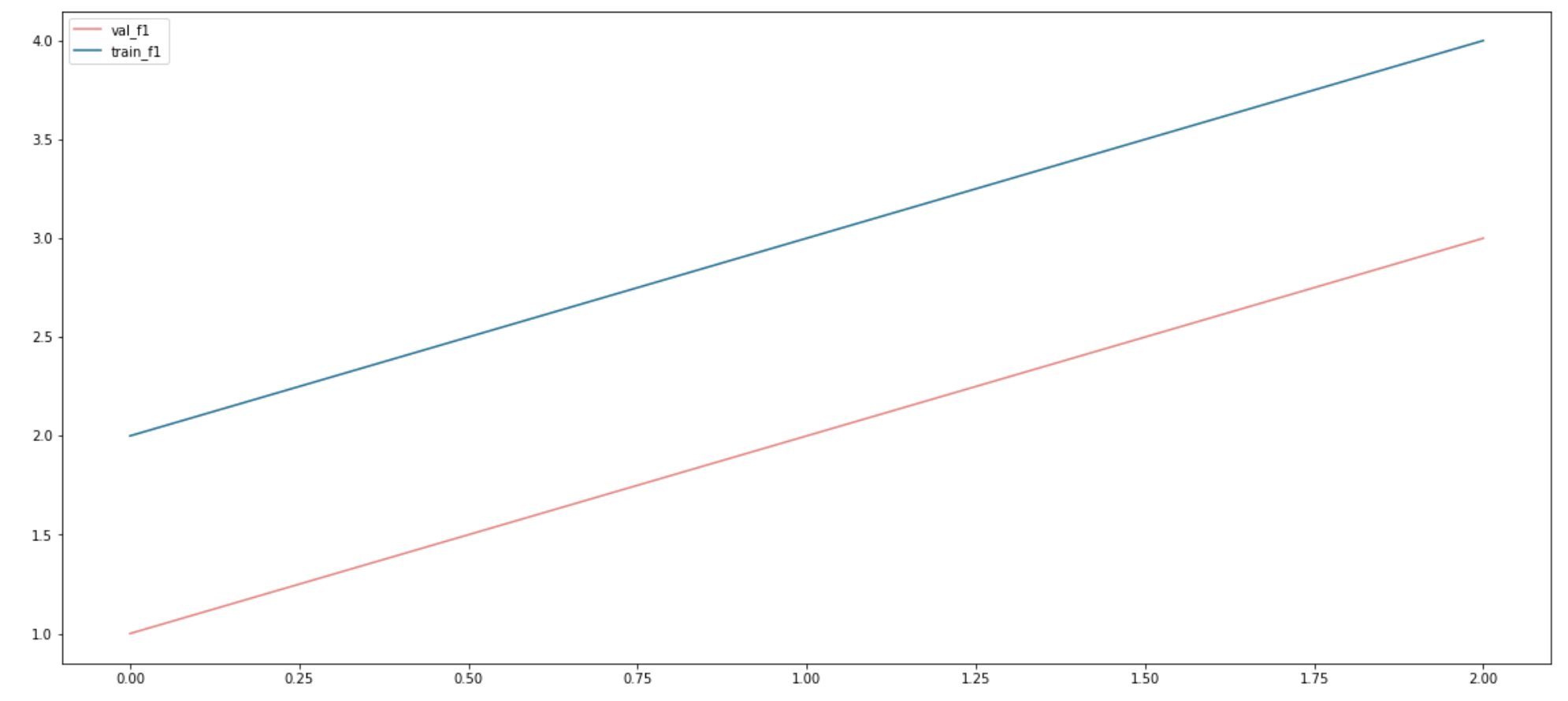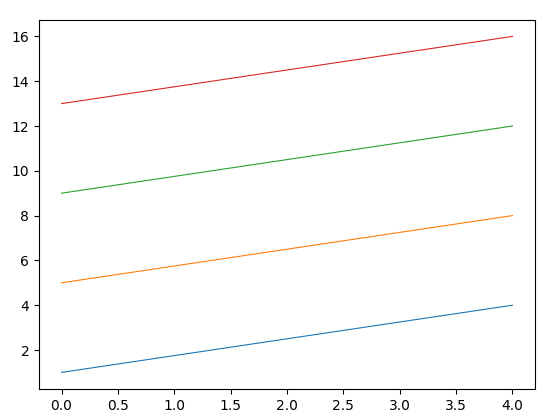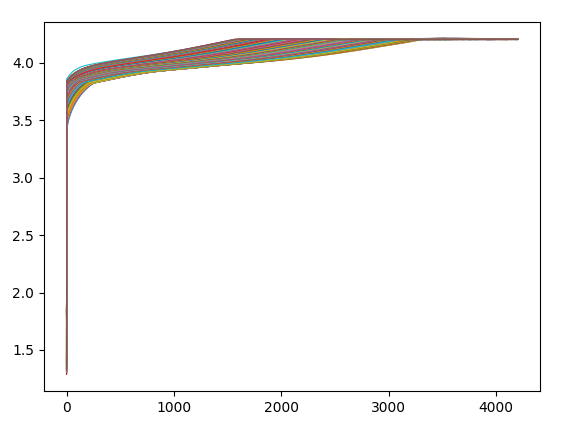• 案例：芝麻信用失信用户分析 关于pie函数的参数我们讲了这么多，光讲不练假把式，我们接下来通过案例，来绘制个个性化的...import matplotlib.pyplot as plt # 设置绘图的主题风格（不妨使用R中的ggplot分隔） pl

pie函数参数解读

plt.pie(x, explode=None, labels=None, colors=None,
labeldistance=1.1, startangle=None,
textprops=None, center=(0, 0), frame=False)

x：指定绘图的数据；

explode：指定饼图某些部分的突出显示，即呈现爆炸式；

labels：为饼图添加标签说明，类似于图例说明；

colors：指定饼图的填充色；

autopct：自动添加百分比显示，可以采用格式化的方法显示；

pctdistance：设置百分比标签与圆心的距离；

labeldistance：设置各扇形标签（图例）与圆心的距离；

startangle：设置饼图的初始摆放角度；

counterclock：是否让饼图按逆时针顺序呈现；

wedgeprops：设置饼图内外边界的属性，如边界线的粗细、颜色等；

textprops：设置饼图中文本的属性，如字体大小、颜色等；

center：指定饼图的中心点位置，默认为原点

frame：是否要显示饼图背后的图框，如果设置为True的话，需要同时控制图框x轴、y轴的范围和饼图的中心位置；

案例参考：芝麻信用失信用户分析

关于pie函数的参数我们讲了这么多，光讲不练假把式，我们接下来通过案例，来绘制一个个性化的饼图。关于绘图数据，我们借用芝麻信用近300万失信人群的样本统计数据，该数据显示，从受教育水平上来看，中专占比25.15%，大专占比37.24%，本科占比33.36%，硕士占比3.68%，剩余的其他学历占比0.57%。对于这样一组数据，我们该如何使用饼图来呈现呢？

import matplotlib.pyplot as plt

# 设置绘图的主题风格（不妨使用R中的ggplot分隔）
plt.style.use('ggplot')

# 构造数据
edu = [0.2515,0.3724,0.3336,0.0368,0.0057]
labels = ['中专','大专','本科','硕士','其他']

explode = [0,0.1,0,0,0]  # 用于突出显示大专学历人群
colors=['#9999ff','#ff9999','#7777aa','#2442aa','#dd5555'] # 自定义颜色

# 中文乱码和坐标轴负号的处理
plt.rcParams['font.sans-serif'] = ['Microsoft YaHei']
plt.rcParams['axes.unicode_minus'] = False

# 将横、纵坐标轴标准化处理，保证饼图是一个正圆，否则为椭圆
plt.axes(aspect='equal')

# 控制x轴和y轴的范围
plt.xlim(0,4)
plt.ylim(0,4)

# 绘制饼图
plt.pie(x = edu, # 绘图数据
explode=explode, # 突出显示大专人群
labels=labels, # 添加教育水平标签
colors=colors, # 设置饼图的自定义填充色
autopct='%.1f%%', # 设置百分比的格式，这里保留一位小数
pctdistance=0.8,  # 设置百分比标签与圆心的距离
labeldistance = 1.15, # 设置教育水平标签与圆心的距离
startangle = 180, # 设置饼图的初始角度
counterclock = False, # 是否逆时针，这里设置为顺时针方向
wedgeprops = {'linewidth': 1.5, 'edgecolor':'green'},# 设置饼图内外边界的属性值
textprops = {'fontsize':12, 'color':'k'}, # 设置文本标签的属性值
center = (1.8,1.8), # 设置饼图的原点
frame = 1 )# 是否显示饼图的图框，这里设置显示

# 删除x轴和y轴的刻度
plt.xticks(())
plt.yticks(())
# 添加图标题
plt.title('芝麻信用失信用户教育水平分布')

# 显示图形
plt.show()转载自公众号：“每天进步一点点2015”，

展开全文数据可视化 matplotlib 数据分析
• 今天小编就为大家分享篇解决python中用matplotlib画多幅图时出现图形部分重叠的问题，具有很好的参考价值，希望对大家有所帮助。一起跟随小编过来看看吧
• matplotlib一图画两线

千次阅读 2019-11-01 10:30:15
import matplotlib.pyplot as plt val_f1_list=[1,2,3] train_f1_list=[2,3,4] plt.figure(figsize=[20,9]) plt.plot(val_f1_list,label="val_f1",color="#F08080") plt.plot(train_f1_list,label="train_f1",color....
import matplotlib.pyplot as plt
val_f1_list=[1,2,3]
train_f1_list=[2,3,4]
plt.figure(figsize=[20,9])
plt.plot(val_f1_list,label="val_f1",color="#F08080")
plt.plot(train_f1_list,label="train_f1",color="#0B7093")
plt.legend()
plt.show()展开全文• 我们的需求是把该表中的所有行看做条曲线并在一张中绘制出所有的曲线。 1 2 3 4 5 6 7 8 9 10 11 12 13 14 15 16 代码 一般的绘图, 在曲线条数比较少时, 只...

需求

有一数据集如下表所示, 它是一组二维数据, 每行代表了一条曲线。 我们的需求是把该表中的每行看做一条曲线, 并在一张图中绘制出所有的曲线,也即所有行数据。

 1 2 3 4 5 6 7 8 9 10 11 12 13 14 15 16

代码

一般的绘图, 在曲线条数比较少时, 只需要多添加几个 plot 语句即可。但是在曲线条数动辄数百上千时, 我们使用手动的 plot 添加效率十分低下(查过一些资料, 循环执行 plot 语句是不奏效的, 它会弹出 n 个图像框, 每个图像中仅有一条曲线, 所以只有手动添加 plot 语句来实现)。为了防止手动添加 plot 语句的繁琐情况, 我们使用 字符串拼接 + Python执行字符串代码 来实现二维数据图的绘制,  代码如下。

import matplotlib.pyplot as plt
import numpy as np

# 创建二维数据和坐标
x = np.linspace(0, 4, 4)      # 创建x的取值范围 0-20 范围内 20 个点
data = [[1,2,3,4],[5,6,7,8],[9,10,11,12],[13,14,15,16]]
lineSize = len(data) # 获取数据的行数 一维特征

# 生成绘图代码
## 绘图
y1 = x
code = ""
for i in range(lineSize):
code += "plt.plot(x, data[{}], lw=0.8)".format(i) + "\n"  # 拼接图像

print(code,lineSize)
c1 = compile(code, "", mode="exec")     # 将字符串编译为 exec 可执行的类型
exec(code)  # 执行字符串代码
plt.show()  # 打印图像

效果shape = (170,4200) 的二维数据图像, 采用 numpy 作为数据裁剪方式说明

比较庞大的数据集可以使用 numpy 来进行加载和裁剪, matplotlib 支持 ndarray 类型数据的读取和绘制, 代码只需要应用自己的数据集做一些修改即可使用。

感谢大家的阅读, 评论区欢迎您的讨论和建议~~

展开全文python 开发语言 后端
• import matplotlib.pyplot as plt import matplotlib.patches as patches # 根据坐标作图 def plot_pic(): img_path = "000000000650.jpg" txt_path = "000000000650.txt" img = plt.imread(img_path) h,w,c =...
import matplotlib.pyplot as plt
import matplotlib.patches as patches

# 根据坐标作图
def plot_pic():
img_path = "000000000650.jpg"
txt_path = "000000000650.txt"

h,w,c = img.shape

coords = []
for line in txt_lines:
new_line = line.strip().split(" ")[1:]
# [x_center,y_center,width,hieght]坐标转换成[left,top,width,height]
width = float(new_line) * w
height = float(new_line) * h
left = float(new_line) * w - width / 2
top = float(new_line) * h - height / 2

coords.append([left,top,width,height])

fig, ax = plt.subplots(1, 1)
ax.imshow(img)
currentAxis = fig.gca()

#
for index, coord in enumerate(coords):
rect = patches.Rectangle((coord, coord), coord, coord,
linewidth=1, edgecolor='r',facecolor='none')

plt.show()
print(0)
if __name__ == "__main__":
plot_pic()

其中
000000000650.jpg图片如下：000000000650.txt内容如下：

15 0.519398 0.544087 0.476359 0.572061
2 0.501859 0.820726 0.996281 0.332178
#[class x_center y_center width height] 复制请删除这一行

通过运行上面的程序，得到如下结果：rectangle函数的参数解释如下：

Rectangle(xy,width,heigth,angle=0,**kwargs)
参数
xy:2元组，矩形左上角坐标
width：矩形的宽度
height：矩形的高度
angle：float，可选，矩形相对于x轴逆时针旋转角度，默认0
fill：bool，可选，是否填充矩形

展开全文python
• 一幅图两个坐标曲线 :param loss_history: loss列表 :param val_acc_history: acc列表 """ fig = plt.figure() # 第1个坐标轴 ax1 = fig.add_subplot(111) ax1.plot(range(len(loss_history)), lpython 深度学习 matplotlib
• 上Python数据分析课的时候，作业中有要将多个子图放在中，如何来操作呢？ 正文 1、subplot 直接指定划分方式和位置进行绘图 t=np.arange(0.0,2.0,0.1) s=np.sin(t*np.pi) plt.subplot(2,2,1) #要生成两行两列...
• 本示例演示在一个figure对象中画两幅柱状一幅纵向（plt.bar)，一副横向(plt.barh)#导入numpy和matplotlib库>>> import numpy as np>>> import matplotlib.pyplot as plt#生成x轴刻度>>> x=np.arange(6)#生成y轴...
• import matplotlib.pyplot as plt from scipy.io import loadmat mat = loadmat('data1.mat') # 假设文件中有32张图片 img= mat['img'] plt.figure() for i in range(1,X): # X为图片的个数 plt.subplot(a,b,i-1)...python
• 每个pyplot函数对一幅图片(figure)做一些改动：比如创建新图片，在图片创建一个新的作图区域(plotting area)，在一个作图区域内画直线，给添加标签(label)等。matplotlib.pyplot是有状态的，亦即它会保存当前图片...
• 以及如何在一幅图有多个子图的情况下，删除重复的图例？我用一个简单的例子说明一下。 import pandas as pd import numpy as np import matplotlib.pyplot as plt fig = plt.figure(1) ax1 = fig.add_subplot(2,2,1...
• Matplotlib显示多张图片¶概要¶本文主要讲解了如何使用Matplotlib实现多窗口图片显示.keywords subplot matplotlib 多窗口 图片显示subplot的API讲解¶在Matplotlib里面绘制多个，需要使用subplot方法， 具体的...
•python matplotlib 数据挖掘
• 主要介绍了Python matplotlib读取excel数据并用for循环画多个子subplot操作，具有很好的参考价值，希望对大家有所帮助。一起跟随小编过来看看吧
• 在实际的工作中，我们有 种需求是需要在块画布上输出多个相关的图形，进行比较或多角度分析，如下所示： matplotlib和pyplot有当前的图形（figure）和当前的轴 （axes）的概念，所有的作图命令都是对当前的...
• python matplotlib 显示图像

万次阅读 多人点赞 2017-12-29 21:34:47
python matplotlib 显示图像 首先需要importimport os...import matplotlib.pyplot as plt显示一幅彩色图片img = Image.open(os.path.join('images', '2007_000648' + '.jpg'))plt.figure("Image") # 图像窗口名称 pltmatplotlib 显示图片
• 文章目录如何在一张图中绘制子图（仅在竖直方向绘制）二、如何在一张图中绘制子图（仅在水平方向绘制）二、...# 将a1定义为绘图框的子图，211表示将绘图框划分为2行1列,最后的1表示第一幅图 a1 = fig.add_subplot(211python
• matplotlib 多个同时显示个画板上代码效果 代码 import matplotlib.pyplot as plt import numpy as np plt.rcParams['font.sans-serif'] = ['SimHei'] # 步骤（替换sans-serif字体） plt.rcParams['axes....python
• 以下展示一些用 matplotlib 画条形、折线、饼图以及散点的示例，其中类似于图例、坐标轴名称，标题等的显示方法是一样的，不另做介绍。 0、引入模块 import random import matplotlib.pyplot as plt import ...python画图
• . 创建数据 x = [1, 2, 3, 4, 5, 6, 7] y = [1, 3, 4, 2, 5, 8, 6] 二. 绘制大 确定大左下角在整个figure中的位置。假设figure为10x10，创建左下角在(1, 1)、宽为8、高为8的坐标系 left, bottom, width, ...
• 灰度化处理就是将一幅色彩图像转化为灰度图像的过程。彩色图像分为R，G，B三个分量，分别显示出红绿蓝等各种颜色，灰度化就是使彩色的R，G，B分量相等的过程。灰度值大的像素点比较亮（像素值最大为255，为白色），...
• How could I plot the following 3 functions (i.e. sin, cos and the addition), on the domain t, on the same ...from numpy import *import mathimport matplotlib.pyplot as pltt = linspace(0, 2*math.pi,...
• 它的文档相当完备，并且Gallery页面中有上百缩略，打开之后都有源程序。因此如果你需要绘制某种类型的，只需要在这个页面中浏览/复制/粘贴一下，基本上都能搞定。 在Linux下比较著名的数据工具还有gnuplot，...
• 上一节了解了一张画布中只有个子图绘图方法后，现在来讨论一张画布中有多个子图的绘图实践，是实现方式也是分：Pyplot风格，用plot.subplot()函数实现OO风格，用对应子图axes的方法实现pyplot风格范例代码如下所示...
• Matplotlib在一张画布上画多个的两种方法，plt.subplot,plt.subplots。 目录回顾plt.subplots()画法plt.subplot()画法保存 回顾 之前也用过plt.subplots()在一张上画过多个，今天看到用plt.subplot()的画法想...
• Matplotlib个Python 2D绘图库，能够以多种硬拷贝格式和跨平台的交互式环境生成出版物质量的图形，用来绘制各种静态，动态，交互式的图表。 Matplotlib可用于Python脚本，Python和IPython Shell、Jupyter ...python 数据分析 matplotlib
• Matplotlib绘制一幅曲线的基础知识，也可以作为Matplotlib的绘图模板来使用噢！ 1. # 绘制一条sin曲线和一条cos曲线 2. # 步骤如下： 3. 4. # 1 调用程序包 5. import matplotlib as mpl 6. import ...Matplotlib Python绘图
• Matplotlib画各种图

万次阅读 多人点赞 2018-06-28 13:24:54
matplotlib(条形) 看本文前，请看matlplotlib使用教程https://blog.csdn.net/kun1280437633/article/details/80370622，有基础请忽略 、简单垂直条形 案例：直辖市GDP水平 中国的四个直辖市分别为北京...matplot python...